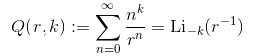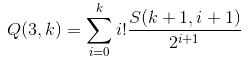# Oligomath 2: When are polylogarithms integers?

Consider the following series, where k and r are positive integers (and r is strictly greater than 1). Here Li_-is a polylogarithm.We shall pose the following question:

For what values of k and r is Q(r, k) an integer?

It is possible to show that Q(r, k) is necessarily rational and that the denominator divides a power of r − 1. We do this by induction on k, expressing Li_-k as a rational function using the following recurrence relation and applying the product rule:In particular, for small values of k, we obtain the following:It is immediately evident that if r = 2, Q(r, k) must necessarily be an integer. On the other hand, if r ≥ 4, Q(r, k) can never be an integer. This leaves only the boundary case of r = 3, which is surprisingly non-trivial.

### Which values of Q(3, k) are integers?

The answer is ‘some of them’.

Recalling that the denominator is necessarily a power of two, we can determine whether Q(3, k) is an integer by examining its 2-adic valuation and seeing whether it is positive. (The 2-adic valuation v2(n) of an integer n is defined to be the largest k such that 2^k divides n. This is extended to rationals by setting v2(p/q) = v2(p) − v2(q).)

I decided to compute v2(Q(3, k)) for all k between 0 and 2047. The results are summarised in the grid below, where non-integers are red, integers are green, and the saturation of the colour corresponds to the absolute value of the 2-adic valuation:There are lots of obvious patterns, but many of them seem to break eventually. In particular, one may initially have conjectured that all numbers of the form 64n + 56 are non-integers, but this pattern breaks down at the pale green square corresponding to 1528. Indeed, there appears to be a ruler sequence of spikes originating from the right-hand side of the grid, which (extrapolated in the natural way) would eventually hit every residue class and therefore break every congruence pattern.

So far, Matei Mandache and Sahl Khan established the following facts:

• If k is a power of two, then Q(3, k) is not an integer;
• If k is one less than a power of two (and k ≥ 3), then Q(3, k) is an integer.

### Stirling numbers of the second kind

Since there seems to be no obvious pattern, we decided to investigate the expression for the polylogarithm in terms of Stirling numbers of the second kind:To compute the 2-adic valuation of a sum, it would certainly help to compute the 2-adic valuation of each term in the sum. It would then be possible to obtain either a lower bound or the exact value* of the 2-adic valuation of the sum by applying the following rules (known to anyone who’s played Gabriella Cirulli’s 2048 game):

• If v2(a) = v2(b), then v2(a + b) > v2(a);
• Otherwise, v2(a + b) = min(v2(a), v2(b)).

* We obtain the exact value if the number of terms of minimal v2 is odd; otherwise, we merely obtain a lower bound.

By multiplicativity, it suffices to know the 2-adic valuations of the following:

• v2(i!) = i − (sum of binary digits of i)
• v2(2^(i+1)) = i + 1
• v2(S(k + 1, i + 1)) = ???

Fortunately for us, there exists a paper on arXiv entitled:

The 2-adic valuations of Stirling numbers of the second kind

Considering the fact that we want to know the 2-adic valuations of Stirling numbers of the second kind, this paper seemed rather promising. However, upon closer inspection, it is by no means exhaustive (many values are not covered by the paper), so is unlikely to answer our question definitively.

So, as usual, let the collaborations begin!

This entry was posted in Oligomath. Bookmark the permalink.

### 5 Responses to Oligomath 2: When are polylogarithms integers?

1.Norman says:

You spelt Gabriele Cirulli’s name wrong.

•apgoucher says:

Sorry, I attended a party last night co-hosted by Professor Béla Bollobás and Gabriella Bollobás, so had the variant spelling in mind when I wrote this article. I shall amend it imminently.

2.Stuart Gascoigne says:

Does your red/green graphic really run from 0-2047?
When I compute the first few values of Q(3,k) I get an integer for k=4, 8, 12,13 which suggests that your grid runs from 1-2048. I’m using your first equation sum(n^k/r^n,n=0->infinity) to get e.g. Q(3,8)=17295.

3.A guardian reader in Cambridge says:

I think you will find that, for a positive integer m, that the sequence

Q(3,2^n – m) x 2^n

converges to something non-zero in Z_2. In particular, the quantities Q(3,2^n – m) for fixed m > 0 will not be integral for sufficiently large n, and any congruence class will have (infinitely many) non-integral values.

4.Bill Gosper says: